Module 3 - Functions and Transformations Introduction | Lesson 1 | Lesson 2 | Lesson 3 | Lesson 4 | Self-Test Self Test List the transformations on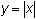in a correct order to produce the graph of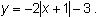Sketch the graph. Check your graph with a calculator. An investment of \$500 is compounded annually at an interest rate of 7%. When will the investment double? Transform the equation 10 = 100(.9897)x into a "zero" problem and solve for x with the Graph screen's Zero feature of your calculator. The quotient rule for logarithms says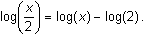Describe the transformation of Y1 = log(x) that produces the graph of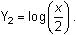A cup containing a hot liquid with an initial temperature of 94° C is set out to cool in a room where the temperature is 23° C. The data in the table below show how the liquid cools. Transform the data to make it exponential and find the exponential regression equation for the transformed data. Shift this equation to fit the original equation. What is the final equation?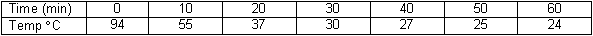Click here to check your answers. < Back ©Copyright 2007 All rights reserved. | Trademarks | Privacy Policy | Link Policy